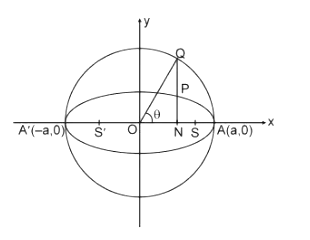# Eccentric Angle and Auxiliary Circle of an Ellipse

A plane curve formed by the intersection of a cone at an angle with respect to the base is an ellipse. It generalises a circle which is a special type of ellipse. The prolongation of an ellipse is termed eccentricity. It ranges from 0 to 1. The general equation of an ellipse is (x2 / a2) + (y2 / b2) = 1. They are similar to other conic sections such as parabolas and hyperbolas. A cross-section of a cylinder that is angled is an ellipse. The definition of an ellipse can also be given in terms of a focal point and directrix, which is the line present on the external side of the ellipse. The ratio of the distance traversed to the focus and distance to the line present outside the ellipse (directrix) is a constant (say k) for all points on the ellipse. The important features of the ellipse are as follows.

1] Eccentricity e = √1 – (b2 / a2)

2] Foci S = (ae, 0) and S’ = (- ae, 0)

3] Equations of directrices: x = a / e and – a / e

4] Principal axis is defined as the major and minor axis of the ellipse taken together.

5] Vertices: The intersection points of an ellipse with the major axis. A (a, 0) and A’ = (- a, 0)

6] Focal chord is the chord that passes through the chord.

7] Double ordinate is the one that is perpendicular to the major axis.

8] Latus rectum is the focal chord that is seen perpendicular to the major axis.

Eccentric Angle and Auxiliary Circle of an Ellipse

The auxiliary circle of an ellipse is the circle that is described on the major axis as diameter. Consider the equation of the ellipse to be (x2 / a2) + (y2 / b2) = 1. The equation of the auxiliary circle will be x2 + y2 = a2. Let Q be a point on the auxiliary circle such that the line through Q that is perpendicular to the x-axis intersects the ellipse at a point P. The points P and Q are corresponding points on the auxiliary circle and the ellipse. The eccentric angle is “θ” of the point P on the ellipse.Parametric Representation of Ellipse

An ellipse with equation (x2 / a2) + (y2 / b2) = 1 is represented in the parametric form by x = a cos θ and y = b sin θ, where θ is the parameter.

P (θ) = (a cos θ, b sin θ) is present on the ellipse then Q (θ) = (a cos θ, a sin θ) is on the auxiliary circle. The range of θ is 0 ≤ θ ≤ 2π.

Example 1: The eccentric angles of the extremities of the latus rectum of the ellipse (x2 / a2) + (y2 / b2) = 1 are given by

A) tan-1 ( ± ae / b)

B) tan-1 (± be / a)

C) tan-1 (± b / ae)

D) tan-1 (± a / be)

Solution: C

The coordinates of any point on the ellipse (x2 / a2) + (y2 / b2) = 1 whose eccentric angle is θ are (a cos θ, b sin θ).

The coordinates of the endpoints of the latus rectum are (ae, ± b2 / a).

∴ a cos θ = ae and b sin θ = ± b2 / a

tan θ = ± b / ae

⇒ θ = tan-1 (± b / ae)

Example 2: Eccentric angle of a point on the ellipse x2 + 3y2 = 6 at a distance 2 units from the centre of the ellipse is

A) π / 4

B) π / 3

C) 3π / 4

D) 2π / 3

Solution: A

Let the eccentric angle of the point be θ, then its coordinates are (√6 cos θ, √2 sin θ).

Hence, 6 cos2 θ + 2 sin2 θ = 4 or cos2 θ = 1 / 2

Hence, cos θ = ± √1 / 2,

θ = π / 4 or 3π / 4

Example 3: The locus of the point of intersection of the perpendicular tangents to the ellipse x2 / 9 + y2 / 4 = 1 is

A) x2 + y2 = 9

B) x2 + y2 = 4

C) x2 + y2 = 13

D) x2 + y2 = 5

Solution: C

The locus of the point of intersection of two perpendicular tangents drawn on the ellipse is x2 + y2 = a2 + b2, which is called the director of the circle.

Given ellipse is x2 / 9 + y2 / 4 = 1.

Locus is x2 + y2 = 13

#### Eccentric Angle and Auxiliary Circle of an Ellipse – Video Lesson